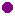Hyperbola as a graph of a function The hyperbola as a graph of the function f:. For the basic set: G = ℚ×ℚ Which of the following statements are correct? To find the solution, move xP on the x-axis and the parameter k.

D: x∈ℚ R: y∈ℚ
D: x∈ℚ , x≠0 R: y∈ℚ , y>0
R: y∈ℚ , y≠0 Arectangle = |k| cm2
 The graph is axisymmetric
 The graph is point symmetric